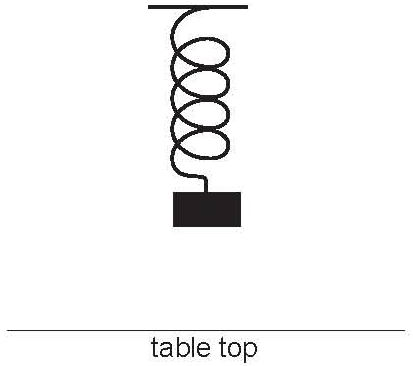# Spring (simple harmonic motion) trig problems

### Spring (simple harmonic motion) trig problems

#### Lessons

• 1.
A mass is supported by a spring so that it rests 50 cm above a table top, as shown in the diagram below. The mass is pulled down to a height of 20 cm above the table top and released at time t = 0. It takes 0.8 seconds for the mass to reach a maximum height of 80 cm above the table top. As the mass moves up and down, its height h, in cm, above the table top, is approximated by a sinusoidal function of the elapsed time t, in seconds, for a short period of time.a)
Graph how the height h of the mass varies with respect to the elapsed time t.

b)
Determine a sinusoidal function that gives the mass's height h above the table top as a function of time t seconds

c)
What is the height of the mass 1.4 seconds after being released?

d)
Find the time t when the mass is 70cm above the table top for the third time?